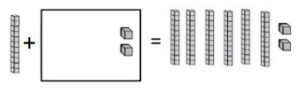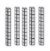# 1st Grade, Activity 272: Use Place Value Understanding and Properties of Operations to Add and Subtract

Given a two-digit number, mentally find 10 more or 10 less than the number, without having to count; explain the reasoning used (Place Value Operations to Add and Subtract).

### Using Models to Find Sums of 10

• Group: Whole Class/Pairs
• Materials: Using Models to Find Sums of 10 sheet

Draw the models and write the example below on the board.Ask: How many base-ten rods can be drawn in the box to get the modeled total?Direct students to start with the total after the equal sign. Next, have students complete the number sentence for
the models as shown below:

10 + _______ (50) + 2 = 62

Lead students to count the rods that they draw by 10 until they reach 50, then have them add the ten outside the
box and 2. Direct them to complete the number sentence as shown below:

10 + 52 = 62

Provide other examples.

After ample practice, issue the Using Models to Find Sums of 10 sheet to each student. Allow students to work in
pairs to complete the sheet. Discuss strategies students used to solve problems.